# Electronics and Communication Engineering - Exam Questions Papers

21.

Consider the following amplifier with negative feedback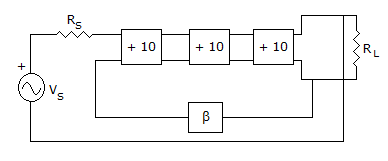If the closed loop gain of above amplifier is + 100, the value of β will be

 A. -9 x 10-3 B. 9 x 10-3 C. -11 x 10-3 D. 11 x 10-3

Explanation:

The T.F.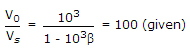103 = 100 - 103 x 100 x β

900 = - 100000 x β

β = - 9 x 10-3.

22.

The signal y(t) = x(- t) is __________ .

 A. causal B. non-causal C. depends on x(t) D. none of these

Explanation:

For signal x(- t) to be causal, the original signal x(t) has to be anticausal.

Similarly for signal x(- t) to be non causal, the original signal x(t) has to be causal.

Hence response of x(t) depends solely on x(t) only.

23.

The autocorrelation function of an ergodic random process is given by __________ .

 A.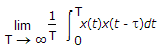B.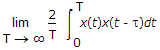C.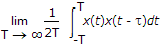D.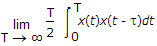Explanation:

The expectations or ensemble average of a random process x(t) are averages "across the process".

The DC value of x(t) is defined by the time average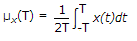The other time average of particular interest is the autocorrelation function Rx(t, T) defined in terms of the sample function x(t) observed over the interval - T ≤ t ≤ T.

Following equation, we may formally define the time-averaged autocorrelation function of a sample function x(t) as follows :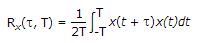This second time-average should also be viewed as a random variable with a mean and variance of its own.

In a manner similar to ergodicity of the mean, we say that the process x(t) is ergodic in the autocorrelation function if the following two limiting conditions are satisfied :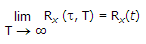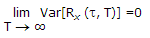.

24.

The ratio of condition current density to displacement current density for a good conductor and a good dielectric is respectively __________ .

 A. greater than 1, greater than 1 B. greater than 1, less than 1 C. less than 1, less than 1

Explanation: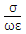= 1 can be considered as the dividing line between the conductor and dielectrics for a good conductor>> 1 and for good dielectrics<< 1 at any frequency ω.

25.

A 'n' bit sequence generator employing SR flip-flop has maximum length of the sequence as :

 A. 2n B. n C. 2n - 1 D. 2n-1